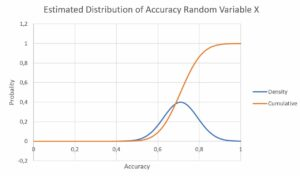Veröffentlicht: von

A method is given which predicts a value for time window W(i).

Posteriori, the quality of the predicted value in the past can be checked against the realized value. A prediction error E(i) and an accuracy measure A(i) can be computed for each window W(i) of the past. We assume that A(i) can be considered as an observation of a random variable X with normal distribution (parameters: expected value „mu“ and square root of its variance „sigma“).

Scenario: The error of prediction value for day n should be tested based on an one-tailed test.

A rough approach to detect that re-training of the prediction model is indicated as follows: The parameters mu and sigma of the assumed normal distribution are estimated by the observations A(1), … A(n-1) of days 1,… n-1

Then the new observation gives the new accuracy A(n) which is checked against the null hypothesis that this value is a realization of the same random variable with identical distribution. If the new value A(n) is too extreme (means that the value drops beneath a specific treshold) the null hypothesis is rejected. This triggers the conclusion that the trained model needs to be updated and a new training cycle is needed.

This algorithm is explained by the following small example (n=6).

 Day Accuracy A(i) previous day W(1) 0,8 previous day W(2) 0,75 previous day W(3) 0,6 previous day W(4) 0,65 previous day W(5) 0,75

Parameter estimation of normal distribution of X:

 mu 0,71 sigma 0,0822Fig: Estimated distribution of X based on the values and assumptions made above.

An acceptance level alpha 0.2 for a one-sided test (left-hand side) would result in a threshold value of

P = 0,641.

That means that error values higher than P= 0,641  would indicate to reject the null hypothesis. If we improve our approach by substituting the estimation of sigma by using the Student’s t-distribution with 4 degrees of freedom the derived threshold t-value for a one-tailed test becomes

P = 0,675 .

If the test method is applied to drift detection in a speed layer the moving time frame leads to recomputed values of mu and sigma for each day. Especially in cases in which a slowly creeping drift occurs it may be considered to introduce a parameter beta which scales the inertness of moving average to achieve a more stable approach:

mu (i) = beta*mu(new) +  (1-beta)*mu(i-1)

sigma (i) = beta* sigma (new) +  (1-beta)* sigma (i-1)